# Simple Interest Questions for Competitive Exams

Simple Interest Questions and Answers with Solution for Competitive Exams. Important and repeated solved questions from the previous year exam question paper of SSC CGL, CHSL, CPO, GD, IBPS Bank, with formula. fundamental and short tricks. Free Online practice of Simple Interest questions for the study of Govt jobs examinations.

Formula
Simple Interest =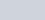$\frac{P\times R\times T}{100}$
P = Principal Amount
R = Rate of Interest per year
T= Time (Number of Years)

### Questions based on Basic Formula of Simple Interest

Q.1: In what time will ₹8000 at 3% per annum produce the same interest as ₹6000 does in 5 years at 4 % simple interest?
a) 5 years
b) 6 years
c) 3 years
d) 4 years

Ans : a) 5 years
Simple Interest for ₹6000 =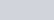$\frac {6000 \times 5 \times 4}{100}$ =₹1200
For ₹8000
Time =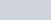$\frac {100 \times 1200}{8000 \times 3}$ = 5 years

Q:2: If a sum of money amounts to ₹12900 and ₹14250 at the end of 4th year and 5th year respectively at a certain rate of simple interest, then the rate of interest is:
a) 10%
b) 12%
c) 18%
d) 20%

Ans : c) 18%
One year Interest = 14250 -12900 = 1350
4 year Interest = 1350×4= 5400
Principal Amount = 12900 – 5400 = 7500
Rate of Interest =$\frac {100 \times 5400}{7500 \times4}$ =18%

Q.3: The sum lent at 5% per annum (i.e.,365 days) simple interest that produces interest, of ₹2.00 per day is :
a) ₹ 1400
b) ₹ 14700
c) ₹ 14600
d) ₹ 7300

Ans : c) ₹ 14600
Total Interest in one year = 365 x2 = 730
P =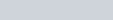$\frac {7300 \times 100}{5\times1} = 14600$

Q.4 : A lent ₹5000 to B for 2 years and ₹3000 to C for 4 years on simple interest at the same rate of interest and received ₹2200 in all from both as interest. The rate of interest per annum is:
a) 7%
b) 5%
c) 8%
d) 10%

Ans : d) 10%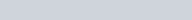$\frac {5000\times2\times R}{100} + \frac {3000\times 4 \times R}{100} = 2200$
=> R = 10%

Q.5: A sum of money at some rate of simple interest amounts to ₹2900 in 8 years and to ₹3000 in 10 years. The rate of interest per annum is :
a) 4%
b) 3%
c) 2.5%
d) 2 %

Ans: d) 2 %
Interest of two years = 3000-2900 =100
Interest in 10 years = 500
Principal = 3000 – 500 = 2500
Rate =$\frac {100 \times 400}{2500\times8}$ = 2%

### Principal amount becomes n Times of Itself in t Years at a Certain Rate of Simple Interest

Q.6: A sum amounts to double in 8 years by simple interest. Then the rate of simple interest per annum is :
a) 10%
b) 12.5%
c) 15%
d) 20%

Ans : b) 12.5%
Simple Interest is equal to P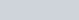$P = \frac {P\times R \times 8}{100}$
R=> 12.5%

Q.7: A sum of money at simple interest triples itself in 15 years. It will become 5 times of itself in :
a) 40 years
b) 36 years
c) 30 years
d) 25 years

Ans : c) 30 years
Simple Interest in 15 years = 3P-P = 2P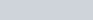$2P = \frac {P \times R \times 15}{100}$
=>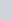$\frac {40}{3}$%
For Amount become 5 times, SI = 4P
4P =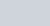$\frac {P\times \frac {40}{3} \times T}{100}$
=> T = 30 years

Q.8: The simple interest on a sum for 5 years is one fourth of the sum. The rate of interest per annum is :
a) 5%
b) 6%
c) 4%
d) 8%

Ans : a) 5%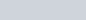$\frac 14 P =\frac {P\times R\times 5}{100}$
R = 5%

### Difference and Equality of Simple Interest Rate and Years

Q.9: A person deposited ₹500 for 4 years and ₹600 for 3 years at the same rate of simple interest in a bank. Altogether he received ₹190 as interest. The rate of simple interest per annum was
a) 4%
b) 5%
c) 2%
d) 3%

Ans : b) 5%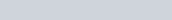$\frac {500 \times R \times 4}{100} + \frac {600 \times R \times 3}{100} = 190$
20R + 18R =190 => R = 5%

Q.10: The difference between the simple interest received from two different banks on ₹500 for 2 years is ₹2.50. The difference between their (per annum) rate of interest is :
a) 0.10%
b) 0.25%
c) 0.50%
d) 1.00%

Ans : b) 0.25%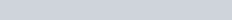${500\times R1 \times 2}{100} - \frac {500 \times R2 2}{100} =2.5$
R1 – R2 = 0.25%

### Simple Interest Ratios : Questions for Competitive Exams

Q.11: If the ratio of principal and the simple interest for 5 years is 10 : 3, then the rate of interest is :
a) 5%
b) 6%
c) 8%
d) 3%

Ans : b) 6%
Ratio = 10 : 3, Principal = 10P, Interest 3P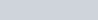$3P = \frac {10P \times R \times 5}{100}$
=> R = 6%

### Increase/decrease in Rate of Interest

Q.12: ₹ 800 amounts to ₹ 920 in 3 years at simple interest. If the interest rate is increased by 3%, it would amount to :
a) ₹ 1056
b) ₹ 1112
c) ₹ 1182
d) ₹ 992

Ans : d) ₹ 992
Simple Interest in 3 years = 920 -800 = 120$120 = \frac {800 \times R \times 3}{100}$
Rate of Interest (R) = 5%
New Rate of Interest = 5+3 = 8%
Simple Interest =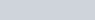$\frac {800\times 8 \times 3}{100} = 192$
Amount become = 800 + 192 =₹ 992

Q.13: A sum of money was invested at a certain rate of simple interest for 2 years. Had it been invested at 1% higher rate, it would have fetched ₹ 24 more interest. The sum of money is :
a) ₹ 1200
b) ₹ 1050
c) ₹ 1000
d) ₹ 9600

Ans : a) ₹ 1200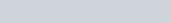$\frac {P\times (R+1)\times 2}{100} - \frac {P\times R \times 2}{100} = 24$
P = ₹ 1200

Q.14: John invested a sum of money at an annual simple interest rate of 10%. At the end of four years the amount invested plus interest earned was ₹ 770. The amount invested was :
a) ₹ 650
b) ₹ 350
c) ₹ 550
d) ₹ 500

Simple Interest =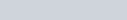$770 - P = \frac {P \times 10 \times 4}{100}$